# Riedtmann classification

(diff) ← Older revision | Latest revision (diff) | Newer revision → (diff)

A finite-dimensional algebraover an algebraically closed fieldis called self-injective if, considered as a right-module, is injective (cf. also Injective module). Well-known examples for self-injective algebras are the group algebras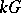for finite groups(cf. also Group algebra). An arbitrary finite-dimensional algebrais said to be representation-finite provided that there are only finitely many isomorphism classes of indecomposable finite-dimensional right-modules.

C. Riedtmann made the main contribution to the classification of all self-injective algebras that are representation-finite. Her key idea was not to look at the algebraitself, but rather at its Auslander–Reiten quiver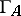. (Quiver is an abbreviation for directed graph, see Quiver.) The vertices of the Auslander–Reiten quiver (see also Representation of an associative algebra) are the isomorphism classes of finite-dimensional-modules. The number of arrows from the isomorphism class ofto the isomorphism class ofis the dimension of the space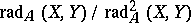, where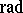is the Jacobson radical of the category of all finite-dimensional-modules. The Auslander–Reiten quiver is a translation quiver, which means that it carries an additional structure, namely a translation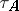mapping the non-projective vertices bijectively to the non-injective vertices. The translation is induced by the existence of almost-spit sequences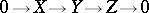(see also Representation of an associative algebra; Almost-split sequence) and sends the isomorphism class of a non-projective indecomposable moduleto the starting term.

The stable part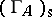of the Auslander–Reiten quiverofis the full subquiver ofgiven by the modules that cannot be shifted into an injective or projective vertex by a power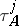for some integer. In [a3], Riedtmann succeeded to prove that for any connected representation-finite finite-dimensionalthe stable partof the Auslander–Reiten quiver is of the shape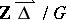, where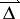is a quiver whose underlying graphis a Dynkin diagram(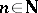),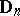(,), or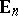(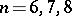) andis an infinite cyclic group of automorphisms of the translation quiver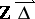. The vertices ofare the pairssuch thatis an integer anda vertex of. Fromto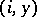there are the arrows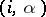withan arrow of. In addition, from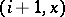tothere exist the arrows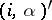with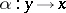an arrow of. The translation maps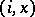to.

For a self-injective algebra, the only vertices of the Auslander–Reiten quiver that do not belong toare the isomorphism classes of the indecomposable projective (and injective) modules. Thus, one can reconstructfromby finding inthe starting points of arrows ofending in projective vertices. These combinatorial data are called a configuration. This shows that for finding all possible Auslander–Reiten quiversof all connected representation-finite self-injective algebrasone has to classify the infinite cyclic automorphism groupsofand the-invariant configurations offor all Dynkin diagrams. For the Dynkin diagrams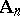andthis classification was carried out in [a4] and [a5].

The classification of the possible configurations for the exceptional Dynkin diagrams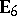,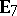,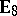turned out to be more difficult. Fortunately, the development of tilting theory offered a convenient way for a solution. Namely, it was observed in [a1] and [a2] that in order to equipwith all possible configurations, one has to form the Auslander–Reiten quivers of the repetitive algebras of the tilted algebras of representation-finite hereditary algebras of type(cf. also Tilted algebra). Nevertheless, the full classification of all these repetitive algebras eventually obtained in [a7] required the use of a computer for handling the huge amount of structures appearing in the case.

If one finally wants to return from the Auslander–Reiten quiverto the algebraitself, one considers the factor of the free-linear category ofby the mesh relations induced by the almost-split sequences. This factor is called the mesh category of. Forming the endomorphism algebra of the direct sum of all projective objects in this mesh category yields(up to Morita equivalence), provided thatis standard (i.e. the mesh category is equivalent to the category of indecomposable finite-dimensional-modules). Non-standard algebras appear only if the characteristic of the fieldisandis of type. They were classified in [a6] and [a9].

It is worth noting that the approach using repetitive algebras was generalized in order to classify the representation-tame self-injective standard algebras of polynomial growth in [a8]. In this case tilted algebras of representation-tame hereditary and canonical algebras replace the tilted algebras of representation-finite hereditary algebras.

How to Cite This Entry:
Riedtmann classification. Encyclopedia of Mathematics. URL: http://encyclopediaofmath.org/index.php?title=Riedtmann_classification&oldid=14608
This article was adapted from an original article by Peter DrÃ¤xler (originator), which appeared in Encyclopedia of Mathematics - ISBN 1402006098. See original article